### Day 11 - Multiplying Monomials - 01.22.15

• New Seats! Pick a different pod!
• Grading for the Functions Test will be completed today!
• Post-Assessment Reflection
• Discuss Pacing of the Class
• Work Days
• If you want something gone over in class, ask. If you are too nervous to ask or request something, email me.
• Question Formulation Discussion

Bell Ringer

1. Which of the following represents the expression written using exponents: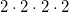?

1.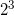2.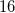3.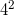4.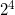5. none of the above

2. Which of the following represents the expression written using exponents: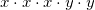?

1.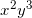2.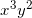3.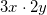4.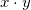5. none of the above

3. Which of the following represents  the expression written using factors: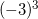?

1.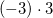2.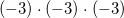3.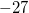4.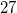5. none of the above

4. Which of the following represents the reciprocal of?

1.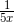2.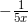3.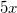4.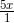5. none of the above

5. What is the definition of monomial?

1. one polynomial

2. number, variable, or a product of a number and a variable(s)

3. an expression with multiple terms

4. an expression with 1 or more terms

5. none of the above

Review
• Prerequisites
• Exponents
• Relationship to multiplication
• Factors
• Reciprocal

Lesson
• Brainstorming Activity
• Monomials
• Multiplying Monomials
• Simplifying Monomial Expressions

Exit Ticket
• Posted on board at the end of the block
Lesson Objective(s)
• How are monomials simplified using multiplication?

#### In-Class Help Requests

Standard(s)
• CC.9-12.A.APR.1 Perform arithmetic operations on polynomials. Understand that polynomials form a system analogous to the integers, namely, they are closed under the operations of addition, subtraction, and multiplication; add, subtract, and multiply polynomials.
• CC.6.EE.1 Apply and extend previous understandings of arithmetic to algebraic expressions.  Write and evaluate numerical expressions involving whole-number exponents.
• CC.9-12.N.RN.1 Extend the properties of exponents to rational exponents. Explain how the definition of the meaning of rational exponents follows from extending the properties of integer exponents to those values, allowing for a notation for radicals in terms of rational exponents. For example, we define 5^(1/3) to be the cube root of 5 because we want [5^(1/3)]^3 = 5^[(1/3) x 3] to hold, so [5^(1/3)]^3 must equal 5.

Mathematical Practice(s)
• #1 - Make sense of problems and persevere in solving them
• #2 - Reason abstractly and quantitatively
• #7 - Look for and make use of structure

Past Checkpoints
• N/A